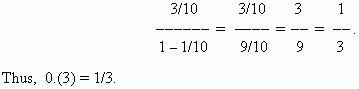# Arithmetic and geometric progressions

Sequences. Numerical sequences. General term of numerical sequence.
Arithmetic progression. Geometric progression. Infinitely decreasing
geometric progression. Converting of repeating decimal to vulgar fraction.

Sequences. Let’s consider the series of natural numbers: 1,  2,  3, … , n – 1, n , … .
If  to replace each natural number n in this series by some number u n , subordinated to some law, we’ll receive a new series of numbers:

u 1 , u 2 , u 3 ,…, u n - 1 , u n , … ,

called a numerical sequence . A number u n is called a general term of the numerical sequence.
E x a m p l e s   of numerical sequences:

2,   4,   6,   8,   10,  … ,  2 n ,  … ;

1,   4,   9,   16,   25,  … , n ² , … ;

1,  1/2,  1/3,  1/4,  1/5,  … , 1/ n , … .

Arithmetic progression. The numerical sequence, in which each next term beginning from the second is equal to the previous term, added with the constant for this sequence number d , is called an arithmetic progression . The number d is called a common difference. Any term of an arithmetic progression is calculated by the formula:

a n =  a 1 + d ( n – 1 ) .

A sum of  n first terms of arithmetic progression is calculated as: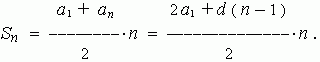E x a m p l e . Find a sum of the first 100 odd numbers.
S o l u t i o n . Use the last formula. Here a 1 = 1, d = 2 . So, we have: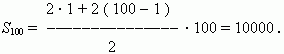Geometric progression. The numerical sequence, in which each next term beginning from the second is equal to the previous term, multiplied by the constant for this sequence number q , is called a geometric progression . The number q is called a common ratio. Any term of a geometric progression is calculated by the formula:

b n =  b 1 q n - 1 .

A sum of n first terms of geometric progression is calculated as: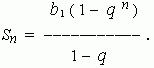Infinitely decreasing geometric progression. This is the geometric progression, with
| q | < 1 . For it the notion of a sum of infinitely decreasing geometric progression is determined as a number, to which a sum of the first n terms  of the considered progression unboundedly approximates at an unbounded increasing of number n . The infinitely decreasing geometric progression sum is calculated by the formula: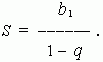E x a m p l e .  Find the sum of the infinitely decreasing geometric progression: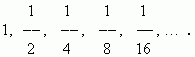S o l u t i o n . Use the last formula. Here b 1 = 1, q = 1/2. So, we have: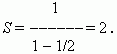Converting of a repeating decimal to a vulgar fraction. Assume, that we want to convert the repeating decimal 0.(3) to a vulgar fraction. Consider this decimal in the more natural form: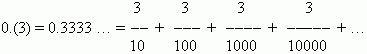This is the infinitely decreasing geometric progression with the first term 3/10 and a common ratio q = 1/10. According to the above shown formula the last sum is equal to: